Select Page

# 12 Science CBSE Answers for MCQ Maths Matrices in Hindi

12 Science CBSE Answers for MCQ Maths Matrices in Hindi to enable students to get Answers in a narrative video format for the specific question.

Expert Teacher provides 12 Science CBSE Answers for MCQ Maths Matrices through Video Answers in Hindi language. This video solution will be useful for students to understand how to write an answer in exam in order to score more marks. This teacher uses a narrative style for a question from Matrices not only to explain the proper method of answering question, but deriving right answer too.

Please find the question below and view the Answer in a narrative video format.

Question:

## Similar Questions from CBSE, 12th Science, Maths, Matrices

Question 1 : If,find the value of x. (View Answer Video)

Question 2 : Compute:. (View Answer Video)

Question 3 : Compute:. (View Answer Video)

Question 4 : Find the value of z,  from the equation:. (View Answer Video)

Question 5 : Given,, find the value of x. (View Answer Video)

### Continuity and Differentiability

Question 1 : Findfor the function. (View Answer Video)

Question 2 : Differentiate the function w.r.t.x. (View Answer Video)

Question 3 : Findfor the function. (View Answer Video)

Question 4 : Differentiate the functionwith respect to x. (View Answer Video)

Question 5 : Differentiate w.r.t.x the function. (View Answer Video)

### Probability

Question 1 : Compute P(A/B) if P(B) =0.5 and P(A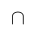B)=0.32. (View Answer Video)

Question 2 : If P(A)=0.8, P(B)=0.5 and=0.4, find. (View Answer Video)

Question 3 : If E and F are two events such that,, Find P(not E and not F). (View Answer Video)

Question 4 : If P(A)=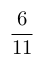, P(B)=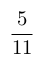and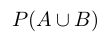=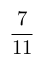, find. (View Answer Video)

### Vector Algebra

Question 1 : Find a vector in the direction of vectorwhich has magnitude 21 units.  (View Answer Video)

Question 2 :  Find the sum of the vectorsand(View Answer Video)

Question 3 :  Ifandare two equal vectors, then write the value of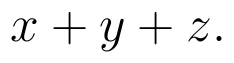(View Answer Video)

Question 4 : Find a unit vector in the direction of(View Answer Video)

Question 5 : Find a vector in the direction ofthat has magnitude 7 units.   (View Answer Video)